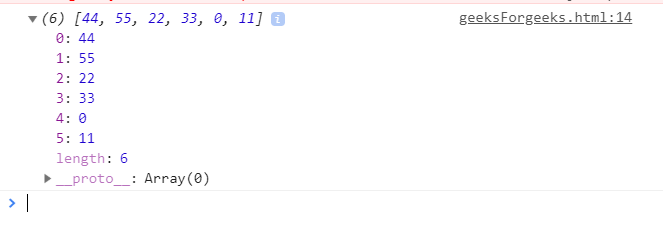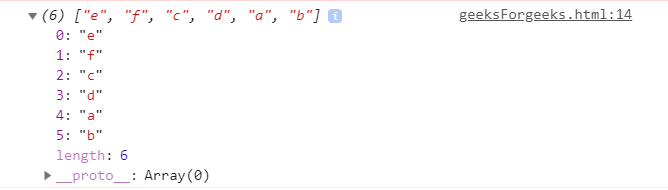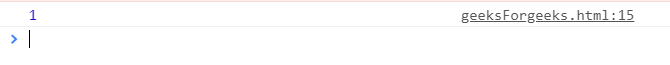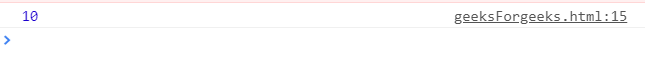Open In App
Related Articles

# Underscore.js _.reduceRight() Function

The _.reduceRight() function is an inbuilt method in Underscore.js which is used to perform operations on each element of the list from the right. When all the elements of the list are passed from right to left to the function/iterate and no more elements remain then the _.reduceRight loop ends. It applies a function simultaneously against two values of the array (from right-to-left) as to reduce it to a single value.

Syntax:

`_.reduceRight(list, function())`

Parameters: It accepts two parameters which are specified below-

• list: It is the list containing some elements which are going to be accessed from right to left.
• function: It is the function which going to perform an operation to reduce the list’s element from right to left.

Return value: It returns the reduced element of the list from right to left.

JavaScript codes to show the working of _.reduceRight() function:

Passing a list of numbers to _.reduceRight() function: The ._reduceRight() function takes the element from the list one by one and do the specified operations on the code. Like here the operation is concatenation of the elements of the list to form a new list. After concatenating all the elements, the reduceRight function ends.

## html

 `<``html``>``  ` `<``head``>``    ``<``script` `type="text/javascript" src ="https://cdnjs.cloudflare.com/ajax/``                      ``libs/underscore.js/1.9.1/underscore-min.js">``    ``<``script` `type="text/javascript" src="https://cdnjs.cloudflare.com/ajax/``                      ``libs/underscore.js/1.9.1/underscore-min.js.map">``    ``<``script` `type="text/javascript" src="https://cdnjs.cloudflare.com/ajax/``                      ``libs/underscore.js/1.9.1/underscore.js">````  ` `<``body``>``    ``<``script` `type="text/javascript">``          ``var list = [[00, 11], [22, 33], [44, 55]];``          ``var answer = _.reduceRight(list, function(a, b)``                          ``{ return a.concat(b); }, []);``          ``document.write(answer);  ``    ``````  ` ``

Output:Passing a list of characters to the _.reduceRight() function: Here also we are doing the same thing as done in the 1st example. The difference is that, the list id not of numbers but rather of characters. So, the final list will contain all the characters but in the right-to-left order of the original list.

## html

 `<``html``>`` ` `<``head``>``    ``<``script` `type="text/javascript" src ="https://cdnjs.cloudflare.com/ajax/``                     ``libs/underscore.js/1.9.1/underscore-min.js">``    ``<``script` `type="text/javascript" src="https://cdnjs.cloudflare.com/ajax/``                     ``libs/underscore.js/1.9.1/underscore-min.js.map">``    ``<``script` `type="text/javascript" src="https://cdnjs.cloudflare.com/ajax/``                     ``libs/underscore.js/1.9.1/underscore.js">```` ` `<``body``>``    ``<``script` `type="text/javascript">``          ``var list = [['a', 'b'], ['c', 'd'], ['e', 'f']];``          ``var answer = _.reduceRight(list, function(a, b)``                           ``{ return a.concat(b); }, []);``          ``document.write(answer);     ``    `````` ` ``

Output:Finding out the value of the last iteration: The ‘num’ variable is a variable that stores the values of the list elements. Therefore since we are returning the value at the end when the function gets over, so, this implies that the list is also over. And since the list is traversed from right to left so the result will be the leftmost element.

## html

 `<``html``>`` ` `<``head``>``    ``<``script` `type="text/javascript" src ="https://cdnjs.cloudflare.com/ajax/``          ``libs/underscore.js/1.9.1/underscore-min.js">``    ``<``script` `type="text/javascript" src="https://cdnjs.cloudflare.com/ajax/``          ``libs/underscore.js/1.9.1/underscore-min.js.map">``    ``<``script` `type="text/javascript" src="https://cdnjs.cloudflare.com/ajax/``          ``libs/underscore.js/1.9.1/underscore.js">```` ` `<``body``>``    ``<``script` `type="text/javascript">``          ``var number=_.reduceRight([1, 2, 3, 4, 5],``                   ``function(memo, num) {``              ``return num;``          ``});``          ``document.write(number);  ``    `````` ` ``

Output:Applying arithmetic operators in the _.reduceRight() function: If we try to perform any arithmetic operation like addition etc on the list of the elements then the first element will be from the Rightmost side.

## html

 `<``html``>`` ` `<``head``>``    ``<``script` `type="text/javascript" src ="https://cdnjs.cloudflare.com/ajax/``                ``libs/underscore.js/1.9.1/underscore-min.js">``    ``<``script` `type="text/javascript" src="https://cdnjs.cloudflare.com/ajax/``                ``libs/underscore.js/1.9.1/underscore-min.js.map">``    ``<``script` `type="text/javascript" src="https://cdnjs.cloudflare.com/ajax/``                ``libs/underscore.js/1.9.1/underscore.js">```` ` `<``body``>``    ``<``script` `type="text/javascript">``          ``var sum=[0, 1, 2, 3, 4].reduceRight(function(a, c ) {``          ``return a + c;``          ``});``          ``document.write(sum); ``    `````` ` ``

Output: